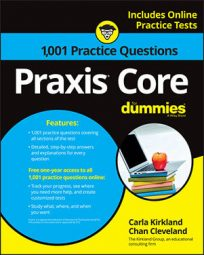##### Praxis Core: 1,001 Practice Questions For DummiesSome problems on the Praxis Core exam will involve systems of equations. Although they look intimidating, they're actually pretty straightforward to solve if you take the right approach.

In the first practice question, you're given two simple equations, and you have to find the solution to the system of equations using elimination. In the second question, you have to use substitution to solve a system of equations, and then answer a separate question based on the values of x and y.

## Practice questions

1. If 2x + 6y = 58 and 5x + 2y = 41, what is the solution to the system of equations?

A. (8, 5) B. (5, 3) C. (–8, 5) D. (4, –3) E. (5, 8)

2. If x + 2y = 22 and 7x – 3y = 1, which of the following numbers is NOT between the values of x and y?

A. 6 B. 8 C. 5 D. 3 E. 7

1. The correct answer is Choice (E).

You can use substitution to solve this system of equations, but elimination is easier. You need to multiply one of the equations by a number that allows you to line up coefficients with the same absolute value for one variable. If you multiply the second equation by –3, you can get the y terms to cancel:

Then you can solve for x:

You can put 5 in for x in either original equation (or any equation derived from them with both variables) to determine the value of y:

The solution is therefore (5, 8). Remember that in an ordered pair, the first number represents x or whichever other variable comes first alphabetically. You can put that solution into any of the equations with both variables and see that it works. None of the other choices work.

2. The correct answer is Choice (D). Because the first equation has x with an understood coefficient of 1, you may want to use the substitution method to solve this system of equations. Use the first equation to solve for x in terms of y:

Now substitute 22 – 2y in for x in the other equation, 7x – 3y = 1, and solve for y. (Make sure you use the other equation. Using the value of one variable in terms of another in the equation where you found it leads to a dead end.)

You can now put the numerical value of y in for y in either original equation and determine the value of x:

Because you know x is 4 and y is 9, you can determine which choice is not between x and y. The only choice that isn't between 4 and 9 is Choice (D).